Others

# Construction of Regular Developable Bézier Patches

Subjects: Computational Mathematics View times: 242

The modeling of developable surfaces is considered a very important application in plat-metal-based industries. Relating to the purpose, this discussion aims to obtain some formulas for constructing the regular developable Bézier patches, in which each boundary curve must be laid in two parallel planes. The results as follows: We find some formulas of the equation systems that are described by the constant, linear, and quadratic control parameters of the regular developable Bézier patches criteria. The new approach is numerically tested for constructing the regular developable Bézier patches, in which their boundary curves are defined, respectively, by the combination of four, five, and six degrees. Click here! (https://www.mdpi.com/2297-8747/24/1/4).

To construct the regular developable Bézier patches, in which each boundary curve of Bézier curves C1(u) and  C2(u) must be laid in two parallel planes, can use regularity condition   C2(u) = ρ(u) C1(u) with the real scalar ρ(u) > 0. Using different values of the control parameters criteria  ρ(u), we will find some formulas of equation systems  that can construct the family of the regular developable Bézier patches.

In case of the control parameters ρ(u) = α R+ constant, we can find the regular developable Bézier patches of four degrees boundary curves, if we first determine the consecutive control points [p0,p1,p3,p4], [q0,q4] and (q4q0)//(p4p0). Second, we calculate α in the equations system that is formulated by the condition of the developable Bézier patches. Finally, the four variable control points [q1,q3,q2,p2] are calculated by four equations in the system. This method also applies to C1(u) and C2(u) of six degrees. The examples result are shown in Figure 1.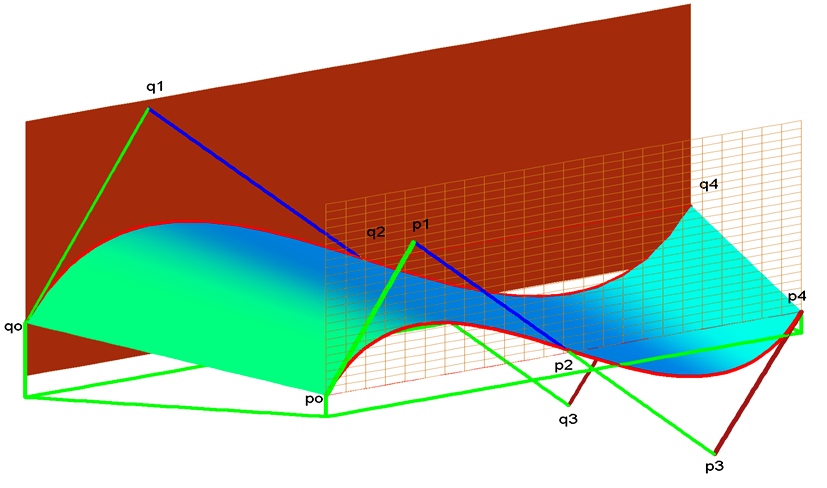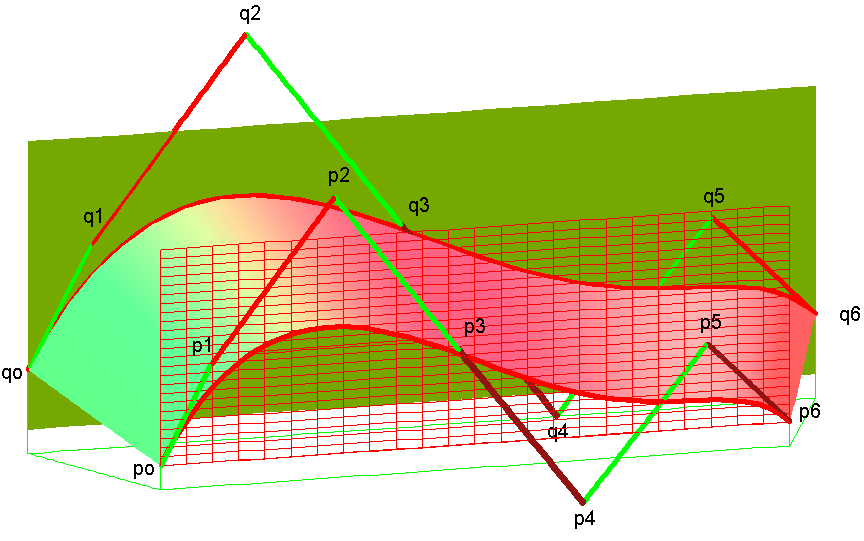(a)                                                                                         (b)

Figure 1. Construction [RDBP(4,4)]C and [RDBP(6,6)]C.

In case of the control parameters ρ(u) = [α0 (1−u) + α1 u] R+ linear, we can find the regular developable Bézier patches of four and five degrees boundary curves, if the points [p0,p1,p3,p4,q0,q5] are fixed. Then, we calculate α0 and α1 in the equations system in which its determinant of the coefficient matrix is different from zero, and we determine the centroid point p of Bézier polygon of the curve C1(u), such that the direction of the vectors (pp0) and (p4p) are in the different sides to the vector (q5q0). Finally, we determine the five control points [q1,q4,p2,q2,q3] of the equations. This method also applies to C1(u) and C2(u) of five and six degrees. The examples result are shown in Figure 2.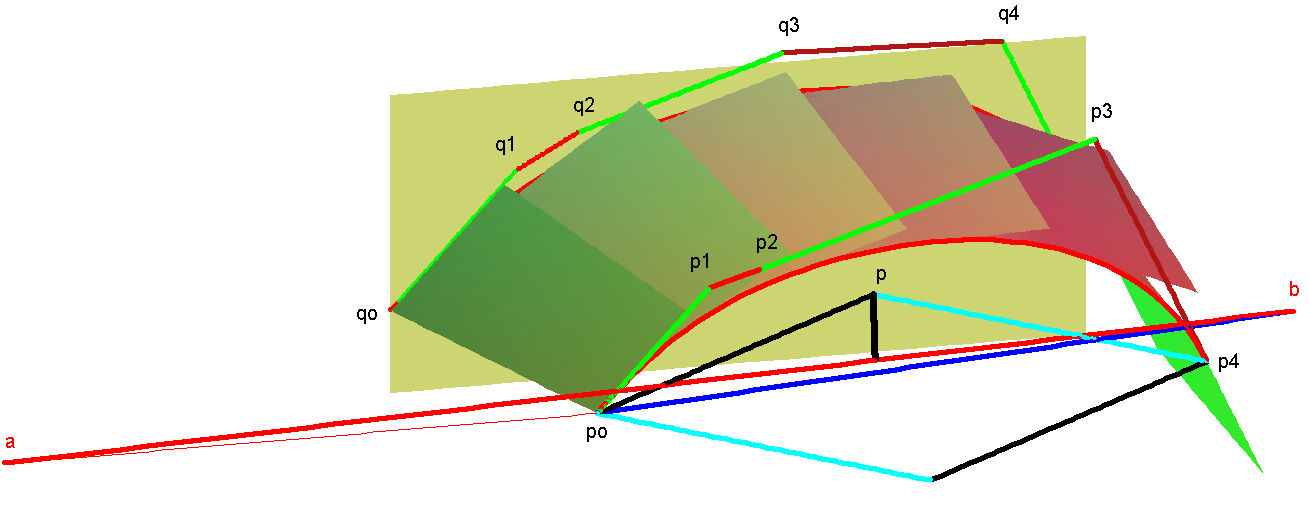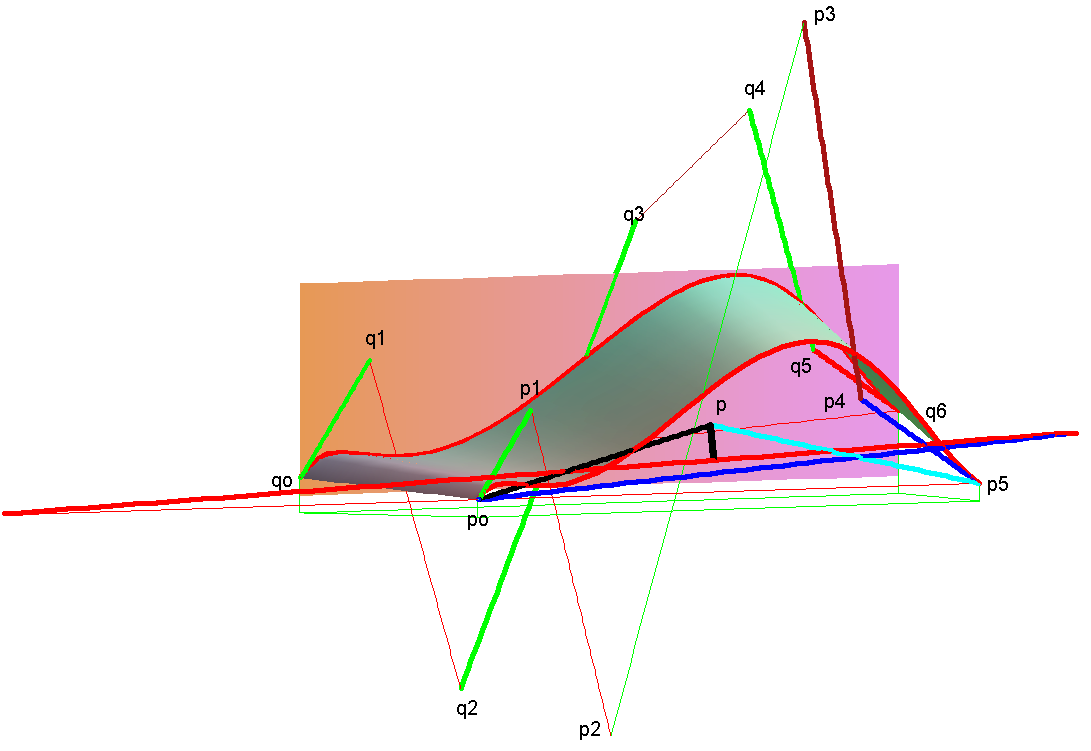(a)                                                                    (b)

Figure 2. Construction [RDBP (4,5)]L and [RDBP(5,6)]L.

In case of the control parameters ρ(u) = [α0 (1−u) + 2α1 (1−u)u + α2 u] R+ quadratic, we can find the regular developable Bézier patches of four and six degrees boundary curves, if the points [p0,p1,p3,p4,q0,q6] are fixed and the elected value α1 verify the equation [(q6q0) − α1v] = α0 (pp0) + α2 (p5p). In this case, we can determine the centroid point p in a position likely in the case of ρ(u) linear. Then, we calculate α0 and α1 in the equations system in which its determinant of the coefficient matrix is different from zero. Finally, we determine the control points [q1,q5,p2,q2,q3,q4] of the equations. The examples result are shown in Figure 3.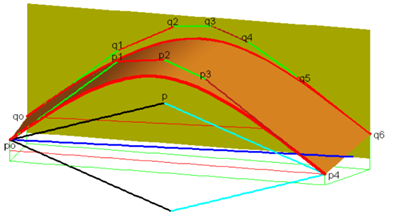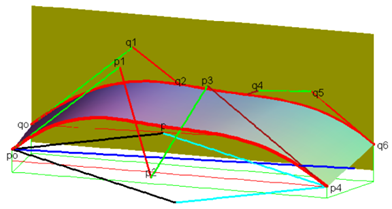(a)                                                                                                        (b)

Figure 3. Construction [RDBP(4,6)]Q.(a) Value α1 = 0;  (b) Value α1 = 0 and position p moved down